• No products in the cart.

# SELF INDUCTION A-LEVEL PHYSICS

##### This Unit is about Self and Mutual Inductance in Physics.

Self Induction

The flux due to the current in the coil links that coil and if the current changes, the resulting flux change induces an emf in the coil itself.

This effect is called self induction. The coil is said to have self inductance, (L) and the coil is said to be an inductor.  The induced emf tends to oppose the growth of current in the coil.

Demonstration  of self induction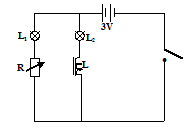L and R have the same resistance. L1 and L2 are identical lamps. When the current is switched on, lamp L2 lights up a second or two later after lamp L1 has lit. This is because the induced emf in  L opposes the current flowing through lamp L2. Therefore the growth of current in L2 to its steady value is delayed.

Variation of current with time through L1 and L2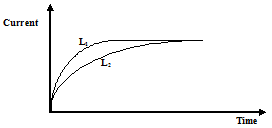If the  3V d.c is replaced by a 3V a.c, the lamp in series with L does not light because the induced emf, due to self induction in L, opposes the applied p.d continuously.

Question: Why L2 light when the iron core is removed in the coil?

Self inductance, L, of a coil is the ratio of the induced emf to the rate at which the current in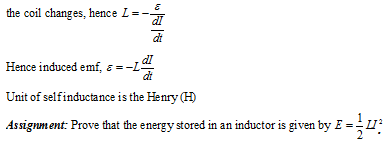THIS VIDEO EXPLAINS MORE ABOUT SELF INDUCTANCE

Mutual Inductance

If two coils i.e. the primary and the secondary coils, are near each other, and  the current in the primary coil is changed, an emf is induced in the secondary coil. This process is called mutual induction.

Demonstration  of mutual  inductionWhen switch is closed, the galvanometer, G, deflects momentarily,  indicating flow of current in secondary coil. This is because the current flowing through the primary coil induces a magnetic field which links up with the secondary coil. When this magnetic field changes, an emf is induced in the secondary coil.

When the switch is opened, the galvanometer deflects momentarily  in the opposite direction. This because the induced flux reduces to zero and hence an emf is induced in a direction according to Lenz’s law.

Mutual inductance, M between two coils is the ratio of the induced emf in the secondary coil to the rate of change of current in the primary coil.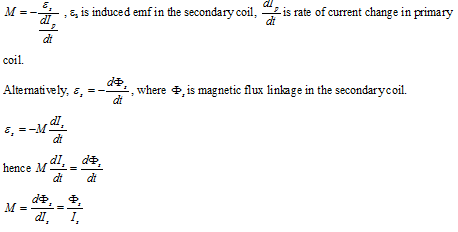Hence mutual inductance is the magnetic flux linkage in the secondary coil when the primary current is 1A.

Unit of mutual inductance is the Henry(H).

Mutual inductance of a solenoid and a coil.

Consider a short secondary coil of Ns turns , area A wound round the middle of a long primary solenoid of n turns per metre.Calibration of a Ballistic galvanometer using a standard mutual inductance

A known mutual inductance can be used to calibrate a ballistic galvanometer so that its sensitivity C can be known.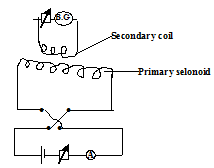THIS VIDEO IS ABOUT MUTUAL INDUCTANCE